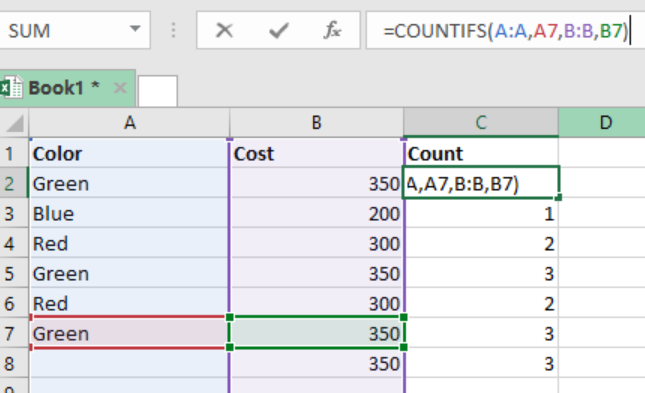Get instant live expert help with Excel or Google Sheets“My Excelchat expert helped me in less than 20 minutes, saving me what would have been 5 hours of work!”

#### Post your problem and you’ll get Expert help in seconds.

Your message must be at least 40 characters
Our professional Expert are available now. Your privacy is guaranteed.

# How to Get First Name from Name with Comma in Excel

While working with excel spreadsheets, a times comes when we are required to get the first name from full name. In most cases, the name format is written as “Last, First” name. To get the first name, we need to use a formula that used the RIGHT, LEN and FIND functions. This article shall provide a clear guide on how one can get the first name from name with comma in excel.Figure 1: How to get the first name from name with comma

## Syntax of the formula

`=RIGHT(name, LEN(name) – FIND(“,”, name) -1)`

Where;

• Name- refers to the full name written in the format; Last, First

## Understanding the formula

The above formula can help us easily get the first name from a full name.  Note that the first and second name are separated by space and a comma. Read on to find out how this formula works.

## How the formula works

• The formula utilizes the RIGHT function to extract those characters that are on the right side of the name.
• We have the FIND function which helps the formula know the number of figures that need to be extracted. This function locates the position of “,” in the name. In our above example, the function operates as shown below;
`FIND(“,”,B4)// locates position of the comma`
• The position of the space and comma are returned as a number by the FIND function.
• The number returned by the FIND function is then subtracted from the total length of the name. This is as elaborated below;
`LEN(B4)-FIND(“,”// shows the length of the first name +1`
• The one that is added at the end of the result is due to the comma. To get the true length of the first name, we are required to subtract 1 from the result.
• The name is in a reserve order. The RIGHT function can thus simply extract the length of the first name.

## Instant Connection to an Expert through our Excelchat Service

Most of the time, the problem you will need to solve will be more complex than a simple application of a formula or function. If you want to save hours of research and frustration, try our live Excelchat service! Our Excel Experts are available 24/7 to answer any Excel question you may have. We guarantee a connection within 30 seconds and a customized solution within 20 minutes.

### Did this post not answer your question? Get a solution from connecting with the expert.Another blog reader asked this question today on Excelchat:## Subscribe to Excelchat.coAnother blog reader asked this question today on Excelchat: